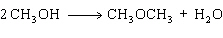Name:    Practice Final B

Multiple Choice
Identify the choice that best completes the statement or answers the question.

1.

Which of the following pairs lists the substance with the higher vapor pressure first?
 A) CCl4 and CBr4 B) C7H16 and C5H12 C) CH3CH2OH and CH3OCH3 D) H2O and H2S

2.

Which of the following is the most polar bond?
 A)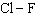B)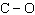C)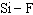D)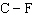3.

Which of the following types of crystalline solids would be expected to have the lowest melting point?
 A) non-polar molecules B) polar molecules C) ionic D) melting point is unpredictable and need more information

4.

Which of the following correctly lists the bond in order of decreasing polarity?
 A)B)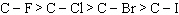C)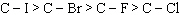D)5.

What is the approximate bond angle in FNO?
 A) 180° B) 109° C) 120° D) 90°

6.

What is the molality of a solution prepared by dissolving 86.9 g of diethyl ether, C4H10O, in 425 g of benzene, C6H6?
 A) 2.76 m B) 2.01 m C) 0.362 m D) 0.498 m

7.

A volatile liquid would
 A) have a low vapor pressure at room temperature B) have weak attractive forces between molecules C) evaporate slowly at room temperature D) have strong attractive forces between molecules

8.

Which of the following kinds of intermolecular forces is found in all molecules?
 A) dipole-dipole interactions B) London forces C) hydrogen bonding D) they are all present in all molecules

9.

Which of the following compounds has the greatest lattice energy?
 A) LiBr (s) B) MgO (s) C) NaI (s) D) CaS (s)

10.

What is the molality of a solution prepared by dissolving 10.0 g of NaCl in 325 g of water?
 A) 30.8 m B) 0.0308 m C) 0.526 m D) 0.000526 m

11.

What is the molecular shape of SCl3F as predicted by the VSEPR theory?
 A) T-shaped B) tetrahedral C) see-saw D) square pyramidal

12.

Diethyl ether has a vapor pressure of 400.0 torr at 18 °C.  When a sample of benzoic acid is dissolved in 500.0 g of diethyl ether, the vapor pressure of the solution is 342 torr.  What is the mole fraction of benzoic acid in solution?
 A) 0.0169 B) 0.0197 C) 0.145 D) 0.855

13.

In which of the following liquids would London forces be the predominant intermolecular force?
 A) H2O B) HF C) ClF D) Br2

14.

Which of the following statements is CORRECT?
 A) a hydrogen bond is an extremely weak dipole-dipole interaction B) hydrogen bonding occurs between any two hydrogen-containing molecules C) London forces are “instantaneous” dipole-dipole interactions D) dipole-dipole interactions occur only between non-polar molecules

15.

What is the hybridization for the Xe in XeF4?
 A) dsp3 B) sp2 C) sp3 D) d2sp3

Problem

16.

Use bond energy data from Table 9.5 in your text to calculate enthalpy for the reaction shown below: